×#### Thank you for registering.

One of our academic counsellors will contact you within 1 working day.

Click to Chat

1800-1023-196

+91-120-4616500

CART 0

• 0

MY CART (5)

Use Coupon: CART20 and get 20% off on all online Study Material

ITEM
DETAILS
MRP
DISCOUNT
FINAL PRICE
Total Price: Rs.

There are no items in this cart.
Continue Shopping• Complete JEE Main/Advanced Course and Test Series
• OFFERED PRICE: Rs. 15,900
• View Details

Practical Geometry Exercise 18.3

Question: 1

Construct a quadrilateral ABCD in which AB = 3.8 cm, BC = 3.4 cm, CD = 4.5 cm, AD = 5 cm and ∠B = 80°.

Solution: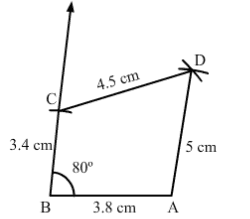Steps of construction:

Step I: Draw AB = 3.8 cm.

Step II: Construct ∠ABC=80°.

Step III: With B as the center and radius 3.4 cm, cut off BC = 3.4 cm.

Step IV: With C as the center and radius 4.5 cm, draw an arc.

Step V: With A as the center and radius 5.3 cm, draw an arc to intersect the arc drawn in Step IV at D.

Step VI: Join AD, BC and CD to obtain the required quadrilateral.

Question: 2

Construct a quadrilateral ABCD, given that AB = 8 cm, BC = 8 cm, CD =10 cm, AD =10 cm and ∠A=45°.

Solution: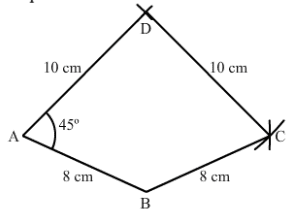Steps of construction:

Step I: Draw AB = 8 cm.

Step III: With A as the centre and radius 10 cm, cut off AD =10 cm.

Step IV: With D as the centre and radius 10 cm, draw an arc.

Step V: With B as the centre and radius 8 cm, draw an arc to intersect the arc drawn in Step IV at C.

Step VI: Join BC and CD to obtain the required quadrilateral.

Question: 3

Construct a quadrilateral ABCD in which AB = 7.7 cm, BC = 6.8 cm, CD = 5.1 cm, AD = 3.6 cm and ∠C=120°.

Solution: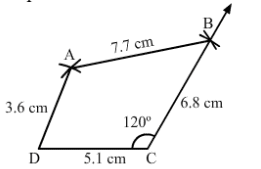Steps of construction:

Step I: Draw DC = 5.1 cm.

Step II: Construct ∠DCB=120°.

Step III: With C as the center and radius 6.8 cm, cut off BC = 6.8 cm.

Step IV: With B as the center and radius 7.7 cm, draw an arc.

Step V: With D as the center and radius 3.6 cm, draw an arc to intersect the arc drawn in Step IV at A.

Question: 4

Construct a quadrilateral ABCD in which AB = BC = 3 cm, AD = CD = 5 cm and ∠B=120°.

Solution: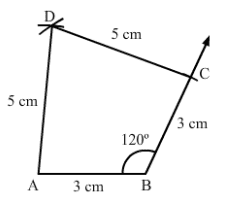Steps of construction:

Step I: Draw AB = 3 cm.

Step II: Construct ∠ABC=120°.

Step III: With B as the center and radius 3 cm, cut off BC = 3 cm.

Step IV: With C as the center and radius 5 cm, draw an arc.

Step V: With A as the center and radius 5 cm, draw an arc to intersect the arc drawn in Step IV at D.

Question: 5

Construct a quadrilateral ABCD in which AB = 2.8 cm, BC = 3.1 cm, CD = 2.6 cm and DA =3.3 cm and ∠A=60°.

Solution: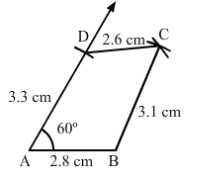Steps of construction:

Step I: Draw AB = 2.8 cm.

Step III: With A as the center and radius 3.3 cm, cut off AD = 3.3 cm.

Step IV: With D as the center and radius 2.6 cm, draw an arc.

Step V: With B as the center and radius 3.1 cm, draw an arc to intersect the arc drawn in Step IV at C.

Step VI: Join BC and CD to obtain the required quadrilateral.

Question: 6

Construct a quadrilateral ABCD in which AB = BC = 6 cm, AD = DC = 4.5 cm and ∠B=120°.

Solution:Steps of construction:

Step I: Draw AB = 6 cm.

Step II: Construct ∠ABC=120°

Step III: With B as the centre and radius 6 cm, cut off BC = 6 cm. Now, we can see that AC is about 10.3 cm which is greater than AD + CD = 4.5 + 4.5 = 9 cm.

We know that sum of the lengths of two sides of the triangle is always greater than the third side but here, the sum of AD and CD is less than AC.

So, construction of the given quadrilateral is not possible.### Course Features

• 728 Video Lectures
• Revision Notes
• Previous Year Papers
• Mind Map
• Study Planner
• NCERT Solutions
• Discussion Forum
• Test paper with Video Solution